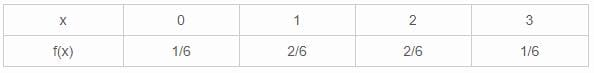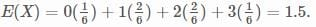# Test: Probability And Expected Value By Mathematical Expectation- 4

## 40 Questions MCQ Test Quantitative Aptitude for CA CPT | Test: Probability And Expected Value By Mathematical Expectation- 4

Description
Attempt Test: Probability And Expected Value By Mathematical Expectation- 4 | 40 questions in 40 minutes | Mock test for CA Foundation preparation | Free important questions MCQ to study Quantitative Aptitude for CA CPT for CA Foundation Exam | Download free PDF with solutions
QUESTION: 1

Solution:
QUESTION: 2

Solution:
QUESTION: 3

### Sum of all probabilities is equal to

Solution:
QUESTION: 4

Let a sample space be S = {X1, X2, X3} which of the fallowing defines probability space on S ?

Solution:
QUESTION: 5

Let P be a probability function on S = {X1 , X2 , X3} if P(X1)= ¼ and P(X3) = 1/3 then P (X2) is equal to

Solution:
QUESTION: 6

The chance of getting a sum of 10 in a single throw with two dice is

Solution:

Option (b) 1 /2 is correct.

Explanation:-

{  There are a total of 36 combinations on throw of two dice.

The sum of 10 could be obtained as :

(6+4, 4+6, 5+5) that is in 3 ways.

Therefore, the required probability here is :-   3 / 36 = 1/ 12
=> 1/ 12

QUESTION: 7

The chance of getting a sum of 6 in a single throw with two dice is

Solution:
QUESTION: 8

P (B/A) defines the probability that event B occurs on the assumption that A has happened

Solution:
QUESTION: 9

The complete group of all possible outcomes of a random experiment given an ________ set of events.

Solution:
QUESTION: 10

When the event is ‘certain’ the probability of it is

Solution:
QUESTION: 11

The classical definition of probability is based on the feasibility at subdividing the possible outcomes of the experiments into

Solution:
QUESTION: 12

Two unbiased coins are tossed. The probability of obtaining ‘both heads’ is

Solution:
QUESTION: 13

Two unbiased coins are tossed. The probability of obtaining one head and one tail is

Solution:
QUESTION: 14

Two unbiased coins are tossed. The probability of obtaining both tail is

Solution:
QUESTION: 15

Two unbiased coins are tossed. The probability of obtaining at least one head is

Solution:
QUESTION: 16

When 3 unbiased coins are tossed. The probability of obtaining 3 heads is

Solution:

Explanation :

Total no. Of outcomes: HHH,  HHT, HTH, THH, TTH, THT, HTT, TTT

No. Outcomes of all heads: 1

Prob. Of all heads = no. Of outcomes of all heads/ total no. Of outcomes

i.e (1/8)

QUESTION: 17

When unbiased coins are tossed. The probability of getting both heads or both tails is

Solution:
QUESTION: 18

Two dice with face marked 1, 2, 3, 4, 5, 6 are thrown simultaneously and the points on the dice are multiplied together. The probability that product is 12 is

Solution:
QUESTION: 19

A bag contain 6 white and 5 black balls. One ball is drawn. The probability that it is white is

Solution:
QUESTION: 20

Probability of occurrence of at least one of the events A and B is denoted by

Solution:
QUESTION: 21

Probability of occurrence of A as well as B is denoted by

Solution:
QUESTION: 22

Which of the following relation is true ?

Solution:
QUESTION: 23

If events A and B are mutually exclusive, the probability that either A or B occurs is given by

Solution:
QUESTION: 24

The probability of occurrence of at least one of the 2 events A and B (which may not be mutually exclusive) is given by

Solution:
QUESTION: 25

If events A and B are independent, the probability of occurrence of A as well as B is given by

Solution:
QUESTION: 26

For the condition P(AB)= P(A)P(B) two events A and B are said to be

Solution:
QUESTION: 27

The conditional probability of an event B on the assumption that another event A has actually occurred is given by

Solution:
QUESTION: 28

In a throw of coin what is the probability of getting tails.

Solution:

Total cases = [H,T] - 2
Favourable cases = [T] -1
So probability of getting tails = 1/2

QUESTION: 29

Demand of products per day for three days are 21, 19, 22 units and their respective probabilities are 0.29, 0.40, 0.35. profit per unit is \$0.50 then expected profits for three days are

Solution:
QUESTION: 30

If P (A)= 1, P(B)= 1, the events A & B are

Solution:
QUESTION: 31

If events A and B are independent then

Solution:
QUESTION: 32

A card is drawn at random from a well-shuffled deck of playing cards. Find the probability that the card drawn is a card of spade or an ace.

Solution:

There are 52 cards in total.

Hence, total Outcomes =52

Probability of the card drawn is a card of spade or an Ace:

Total Aces =4

Number of Aces of Spade =1

Therefore,

Probability of the card drawn is a card of spade or an Ace:

13/52 + 4/52 - 1/52 = 16/52 = 4/13

QUESTION: 33

When a die is tossed, the sample space is

Solution:
QUESTION: 34

Find the expectation of a random variable X?Solution:QUESTION: 35

If events A and B are independent and P(A)= 2/3 , P(B)= 3/5 then P(A+B)is equal to

Solution:
QUESTION: 36

The expected no. of head in 100 tosses of an unbiased coin is

Solution:
QUESTION: 37

A and B are two events such that P(A)= 1/3, P(B) = ¼, P(A+B)= 1/2, than P(B/A) is equal to

Solution:
QUESTION: 38

Probability mass function is always

Solution:
QUESTION: 39

What is the probability of getting an even number when a dice is thrown?

Solution:

The sample space when a dice is rolled, S = (1, 2, 3, 4, 5, and 6)
So, n (S) = 6 E is the event of getting an even number.
So, n (E) = 3
Probability of getting an even number P (E) = Total number of favorable outcomes/Total number of outcomes
n(E) / n(S) = 3/6 = ½

QUESTION: 40

The sum of probability mass function is equal to

Solution:Use Code STAYHOME200 and get INR 200 additional OFF Use Coupon Code